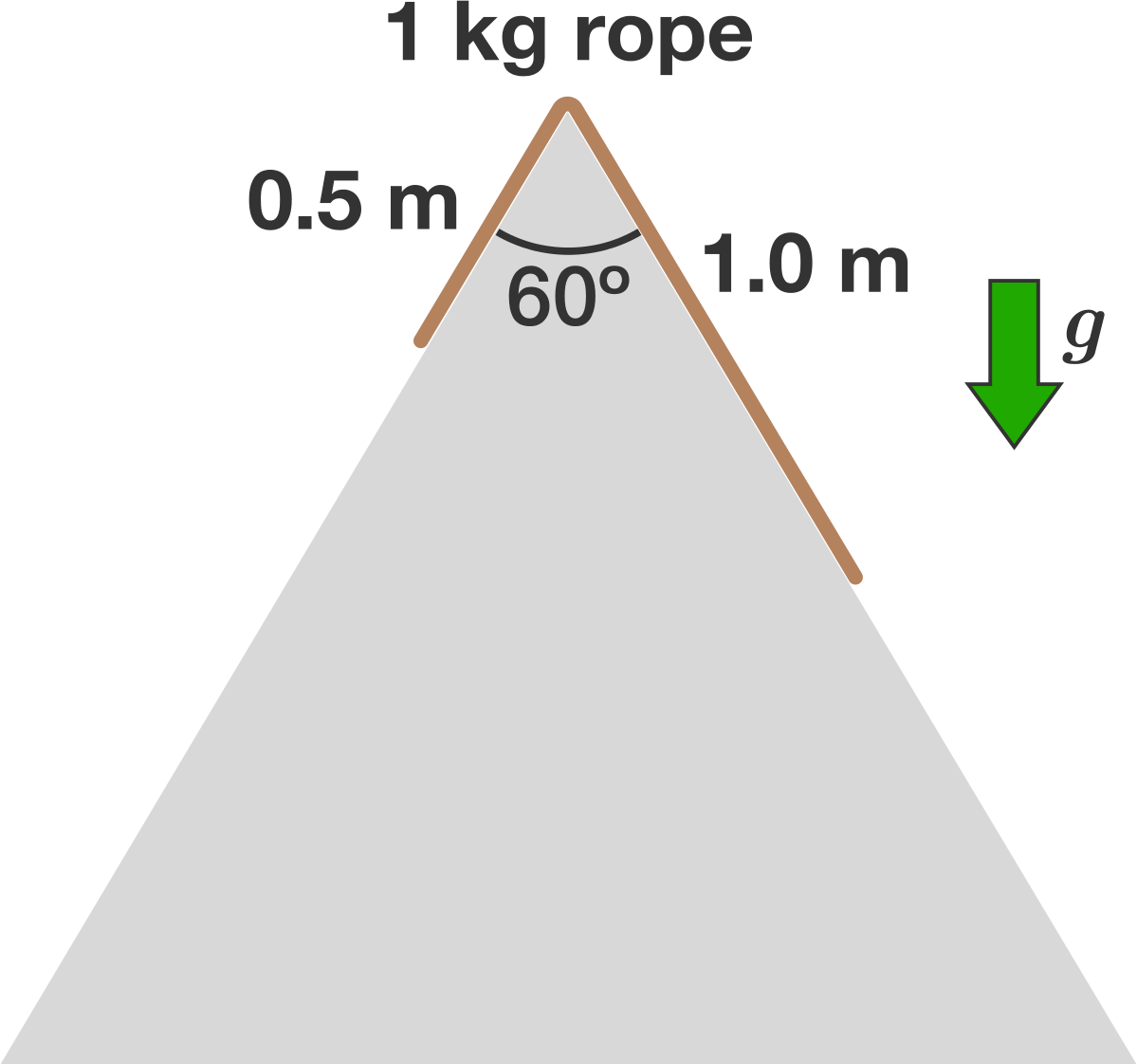Rope sliding on double ramp, how much time would it take before all of the rope is on the right side?

88 views

A 1kg rope of length 1.5m slides down a frictionless double ramp in the shape of an equilateral triangle, as shown in the image below. At time t=0, the rope is at rest with of its length 1m on the right side of the ramp.

How much time elapses before all of the rope is on the right side?

Note: Assume g=10posted May 10, 2017

1kg - 1.5m
? - 1m

Mass of 1m of rope = 1*1/1.5 = 2/3 Kg
Mass of 0.5m of rope = 1- (2/3) = 1/3 Kg

Force on 0.5m Rope = (1/3)*10 = 10/3 N (downward)
Force on 1m Rope = (2/3)*10 = 20/3 N (downward)

Force on 0.5m Rope along the surface = 10/3*cos(30) = 10/3*0.866 = 2.887
Force on 1m Rope along the surface = 20/3*cos(30) = 20/3*0.866 = 5.773

Net force on the rope at any instant
= (5.773 + (10/3)*cos (30)*t) - (2.887 - (10/3)*cos (30)*t)
= (5.773 + 2.887*t) - (2.887 - 2.887*t)
= 2.887 + 5.773*t
Therefore net acceleration on the Rope
= ((2.887 + 5.773*t) N)/(1 Kg) = (2.887 + 5.773*t) ms^(-2)

Distance = (1/2)*acceleration*time^(2)
or D = 0.5*a*t^(2)
Required D is 0.5m of the rope on the left that has to come to the right, therefore time taken for this to happen is

0.5 = 0.5*(2.887 + 5.773*t)*t^(2)
1 = 2.887*t^(2) + 5.773*t^(3)
=> 5.773*t^(3) + 2.887*t^(2) - 1 =0
Solving the cubic equation gives you t = 0.43126s

time = 0.43126 s.answer May 25, 2017

Similar Puzzles

A tank can be filled by the Tap A in 4 Hrs and by Tap B in 6 Hrs. The same tank can be emptied by the Tap C in 8 Hrs. Now suppose we keep all three taps on then how much time it will take to fill?

The ratio between the rates of walking of A and B is 2:3 and therefore A takes 10 minute more than the time taken by B to reach the destination.

If A had walked at double the speed, how much time he would have taken to reach the destination.

+1 vote

If a five-hour candle and a six-hour candle were lit at the same time, how much time would elapse before one candle would be five times longer than the other?